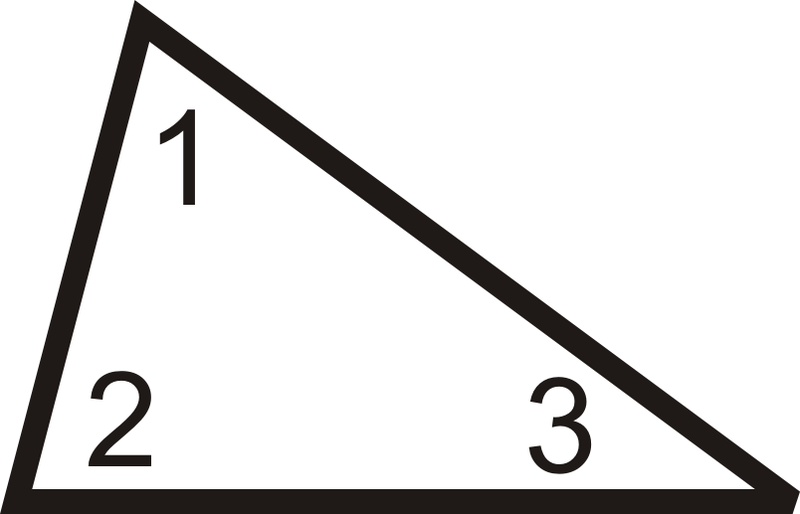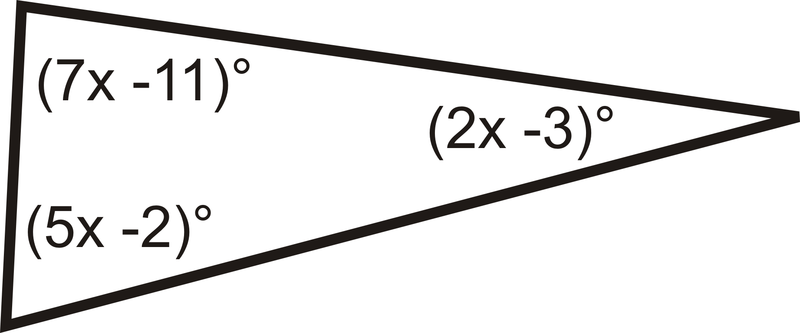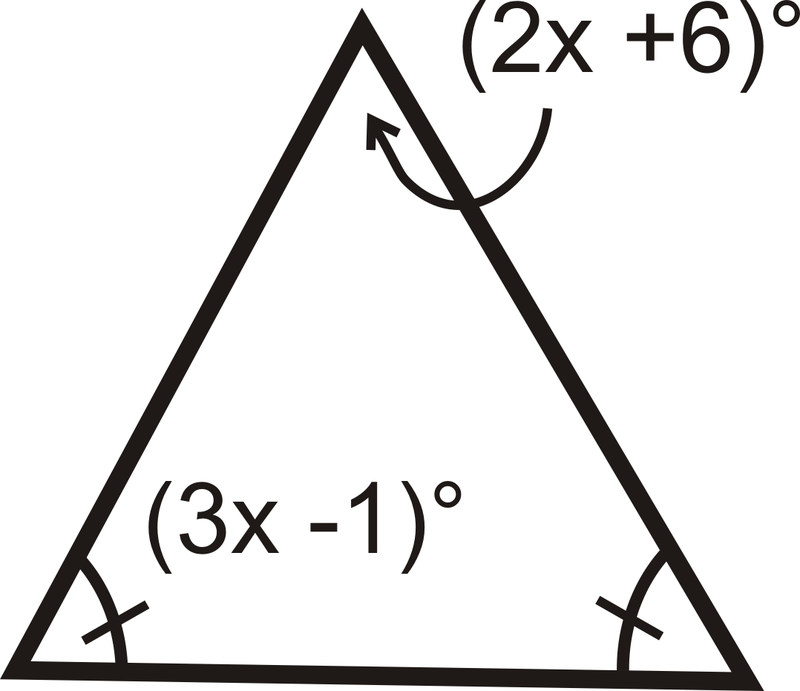# 4.17: Triangle Angle Sum Theorem

•• Contributed by CK12
• CK12

The interior angles of a triangle add to 180 degrees Use equations to find missing angle measures given the sum of 180 degrees.

## Triangle Sum Theorem

The Triangle Sum Theorem says that the three interior angles of any triangle add up to $$180^{\circ}$$.Figure $$\PageIndex{1}$$

$$m\angle 1+m\angle 2+m\angle 3=180^{\circ}$$.

Here is one proof of the Triangle Sum Theorem.Figure $$\PageIndex{2}$$

Given: $$\Delta ABC$$ with $$\overleftrightarrow{AD} \parallel \overline{BC}$$

Prove: $$m\angle 1+m\angle 2+m\angle 3=180^{\circ}$$

Statement Reason
1. $$\Delta ABC with \overleftrightarrow{AD} \parallel \overline{BC}$$ Given
2. \$$angle 1\cong \angle 4,\: \angle 2\cong \angle 5$$ Alternate Interior Angles Theorem
3. $$m\angle 1=m\angle 4,\: m\angle 2=m\angle 5$$ \cong angles have = measures
4. $$m\angle 4+m\angle CAD=180^{\circ}$$ Linear Pair Postulate
5. $$m\angle 3+m\angle 5=m\angle CAD$$ Angle Addition Postulate
6. $$m\angle 4+m\angle 3+m\angle 5=180^{\circ}$$ Substitution PoE
7. $$m\angle 1+m\angle 3+m\angle 2=180^{\circ}$$ Substitution PoE

You can use the Triangle Sum Theorem to find missing angles in triangles.

What if you knew that two of the angles in a triangle measured $$55^{\circ}$$? How could you find the measure of the third angle?

Example $$\PageIndex{1}$$

Two interior angles of a triangle measure $$50^{\circ}$$ and $$70^{\circ}$$. What is the third interior angle of the triangle?

Solution

$$50^{\circ}+70^{\circ}+x=180^{\circ}$$.

Solve this equation and you find that the third angle is $$60^{\circ}$$.

Example $$\PageIndex{2}$$

Find the value of $$x$$ and the measure of each angle.Figure $$\PageIndex{3}$$

Solution

All the angles add up to $$180^{\circ}$$.

\begin{align*} (8x−1)^{\circ}+(3x+9)^{\circ}+(3x+4)^{\circ}&=180^{\circ} \\ (14x+12)^{\circ}&=180^{\circ} \\ 14x&=168 \\ x&=12\end{align*}

Substitute in 12 for $$x$$ to find each angle.

$$[3(12)+9]^{\circ}=45^{\circ} \qquad [3(12)+4]^{\circ}=40^{\circ} \qquad [8(12)−1]^{\circ}=95^{\circ}$$

Example $$\PageIndex{3}$$

What is m\angle T?Figure $$\PageIndex{4}$$

Solution

We know that the three angles in the triangle must add up to $$180^{\circ}$$. To solve this problem, set up an equation and substitute in the information you know.

\begin{align*} m\angle M+m\angle A+m\angle T&=180^{\circ} \\ 82^{\circ}+27^{\circ}+m\angle T&=180^{\circ} \\ 109^{\circ}+m\angle T&=180^{\circ} \\ m\angle T &=71^{\circ}\end{align*}

Example $$\PageIndex{4}$$

What is the measure of each angle in an equiangular triangle?Figure $$\PageIndex{5}$$

Solution

To solve, remember that $$\Delta ABC$$ is an equiangular triangle, so all three angles are equal. Write an equation.

\begin{align*} m\angle A+m\angle B+m\angle C &=180^{\circ} \\ m\angle A+m\angle A+m\angle A&=180^{\circ} \qquad &Substitute,\: all\: angles\: are \: equal. \\ 3m\angle A&=180^{\circ} \qquad &Combine\:like \:terms. \\ m\angle A&=60^{\circ}\end{align*}

If $$m\angle A=60^{\circ}$$, then $$m\angle B=60^{\circ}$$ and $$m\angle C=60^{\circ}$$.

Each angle in an equiangular triangle is $$60^{\circ}$$.

Example $$\PageIndex{5}$$

Find the measure of the missing angle.Figure $$\PageIndex{6}$$

Solution

We know that $$m\angle O=41^{\circ}$$ and $$m\angle G=90^{\circ}$$ because it is a right angle. Set up an equation like in Example 3.

\begin{align*} m\angle D+m\angle O+m\angle G&=180^{\circ} \\ m\angle D+41^{\circ}+90^{\circ}&=180^{\circ} \\ m\angle D+41^{\circ}&=90^{\circ}\\ m\angle D=49^{\circ}\end{align*}

## Review

Determine $$m\angle 1$$ in each triangle.

1.Figure $$\PageIndex{7}$$

2.Figure $$\PageIndex{8}$$

3.Figure $$\PageIndex{9}$$

4.Figure $$\PageIndex{10}$$

5.Figure $$\PageIndex{11}$$

6.Figure $$\PageIndex{12}$$

7.Figure $$\PageIndex{13}$$

8. Two interior angles of a triangle measure $$32^{\circ}$$ and $$64^{\circ}$$. What is the third interior angle of the triangle?

9. Two interior angles of a triangle measure $$111^{\circ}$$ and $$12^{\circ}$$. What is the third interior angle of the triangle?

10. Two interior angles of a triangle measure $$2^{\circ}$$ and $$157^{\circ}$$. What is the third interior angle of the triangle?

Find the value of $$x$$ and the measure of each angle.

11.Figure $$\PageIndex{14}$$

12.Figure $$\PageIndex{15}$$

13.Figure $$\PageIndex{16}$$

14.Figure $$\PageIndex{17}$$

15.Figure $$\PageIndex{18}$$

## Vocabulary

Term Definition
Triangle Sum Theorem The Triangle Sum Theorem states that the three interior angles of any triangle add up to 180 degrees.

Interactive Element

Video: Triangle Sum Theorem Principles - Basic

Activities: Triangle Sum Theorem Discussion Questions

Study Aids: Triangle Relationships Study Guide

Practice: Triangle Angle Sum Theorem

Real World: Triangle Sum Theorem